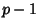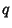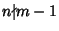## Pollard p-1 Factorization Method

A Prime Factorization Algorithm which can be implemented in a single-step or double-step form. In the single-step version, Primesare found ifis a product of small Primes by finding ansuch thatwhere, witha large number and. Then since,, so. There is therefore a good chance that, in which case(where GCD is the Greatest Common Divisor) will be a nontrivial divisor of.

In the double-step version, a Primescan be factored ifis a product of small Primes and a single larger Prime.

Pollard, J. M. Theorems on Factorization and Primality Testing.'' Proc. Cambridge Phil. Soc. 76, 521-528, 1974.